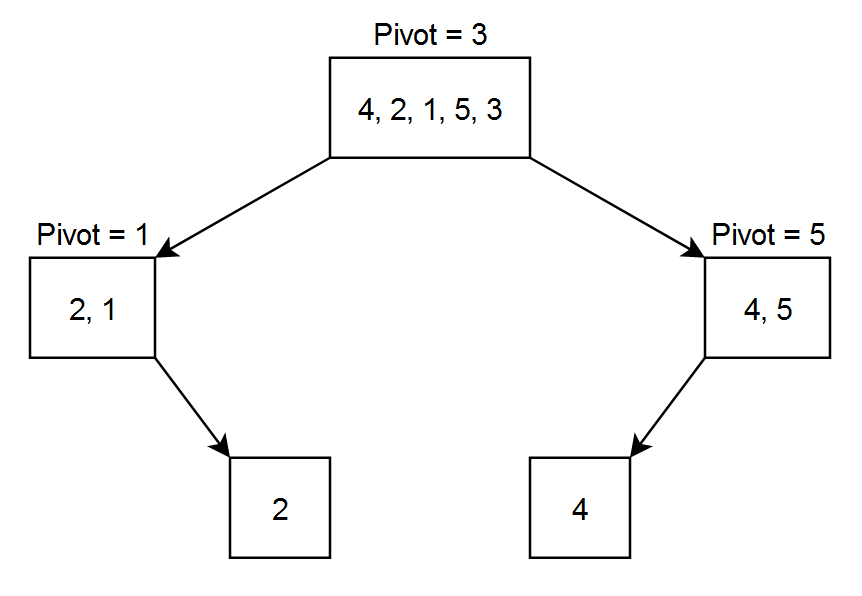New update is available. Click here to update.

# Quick Sort

Contributed by
Anup Kumar Singh
Last Updated: 23 Feb, 2023
Medium0/80
Avg time to solve 10 mins
Success Rate 90 %Share53 upvotes

## Problem Statement

#### Example:

``````Let the array = [ 4, 2, 1, 5, 3 ]
Let pivot to be the rightmost number.
``````#### After the 1st level partitioning the array will be { 2, 1, 3, 4, 5 } as 3 was the pivot. After 2nd level partitioning the array will be { 1, 2, 3, 4, 5 } as 1 was the pivot for the left part and 5 was the pivot for the right part. Now our array is sorted and there is no need to divide it again.

Detailed explanation ( Input/output format, Notes, Images )##### Constraints:
``````1 <= T <= 10
1 <= N <= 10 ^ 3
-10 ^ 9 <= ARR[i] <= 10 ^ 9

Where 'T' is the number of test cases, 'N' is the length of the array 'ARR', and 'ARR[i]' is the array element at index i.

Time limit: 1 sec.
``````
##### Sample Input 1:
``````2
5
4 2 5 1 3
4
6 2 4 1
``````
##### Sample Output 1:
``````1 2 3 4 5
1 2 4 6
``````
##### Explanation of Input 1:
``````Test case 1:
Given array =  [4, 2, 5, 1, 3]
Sorted array = [1, 2, 3, 4, 5]

Test case 2:
Given array =  [6, 2, 4, 1]
Sorted array = [1, 2, 4, 6]
``````
##### Sample Input 2:
``````2
5
5 3 2 6 4
4
1 2 3 4
``````
##### Sample Output 2:
``````2 3 4 5 6
1 2 3 4
``````AutoConsole